Next: 3.2.4 Four-Counter Geometry and Up: 3.2 Measuring the Internal Previous: 3.2.2 The Corrected Asymmetry

## 3.2.3 The Relaxation Function

In the vortex state the muons experience a spatially varying magnetic field due to the periodic arrangement of the vortices. In this case the x-component of the time evolution of the total muon polarization is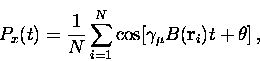(20)
where the sum extends over all muon sites and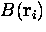is the local field at the site i. Ideally, the sum is replaced by an integral so that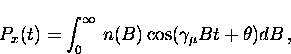(21)
where n(B) is the probability that the muon will precess at the frequency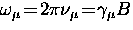. The muon spin polarization Px (t) decays with increasing time because of the inhomogeneous field distribution. Models of the field profile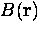for a perfectly ordered vortex lattice will be discussed in the next chapter.

In a real superconductor there are other independent contributions to the variation in the local field. These additional sources of field inhomogeneity can be accounted for by multiplying the muon polarization function by a relaxation function'' G(t) so that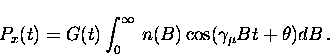(22)
The relaxation function G (t) describes the damping of the muon precession signal which results from the additional sources of field inhomogeneity. One such source is the nuclear dipolar fields in the sample. A Gaussian relaxation function approximately describes the corresponding damping of the muon precession signal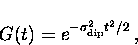(23)
where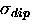is the muon spin depolarization rate'' due to the nuclear dipolar fields.

Signal damping also results from disorder in the vortex lattice. According to Brandt  random disorder can be approximated with a Gaussian relaxation function. The relaxation function which contains the effects of the nuclear dipolar moments and the disorder in the vortex lattice is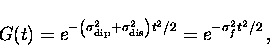(24)
where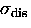is the muon spin depolarization rate due to the lattice disorder and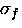is the effective depolarization rate such that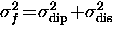.Next: 3.2.4 Four-Counter Geometry and Up: 3.2 Measuring the Internal Previous: 3.2.2 The Corrected Asymmetry